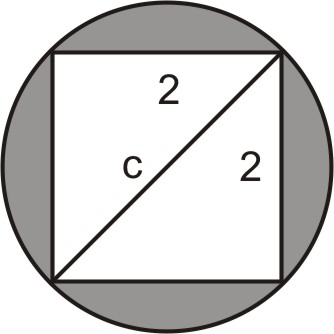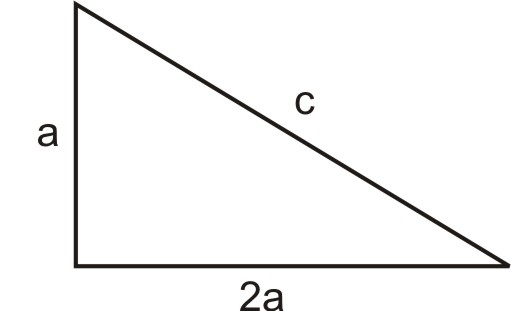# 1.4: Applications Using the Pythagorean Theorem

•• Contributed by CK12
• CK12

Word problems using the Pythagorean Theorem

The Pythagorean Theorem and its converse have many applications for finding lengths and distances.

### Real-World Application: Diagonal Length

Maria has a rectangular cookie sheet that measures 10 inches×14 inches. Find the length of the diagonal of the cookie sheet.

Draw a sketch:Figure $$\PageIndex{1}$$

Define variables: Let $$c= \text{ length of the diagonal}$$.

Write a formula: Use the Pythagorean Theorem: $$a^2+b^2=c^2$$

Solve the equation:

\begin{aligned} 10^2+14^2&=c^2 \\ 100+196&=c^2 \\ c^2&=296 \Rightarrow c=\sqrt{296} \Rightarrow c=2 \sqrt{74} \text{ or } c=17.2 \text{ inches } \end{aligned}

Check: $$10^2+14^2=100+196=296$$ and $$c^2=17.2^2=296$$. The solution checks out.

### Real-World Application: Area of Shaded Regions

Find the area of the shaded region in the following diagram:Figure $$\PageIndex{2}$$

Draw the diagonal of the square in the figure:Figure $$\PageIndex{3}$$

Notice that the diagonal of the square is also the diameter of the circle.

Define variables: Let $$c= \text{ diameter of the circle}$$.

Write the formula: Use the Pythagorean Theorem: $$a^2+b^2=c^2$$.

Solve the equation:

\begin{aligned} 2^2+2^2&=c^2 \\ 4+4&=c^2 \\ c^2&=8 \Rightarrow c=\sqrt{8} \Rightarrow c=2\sqrt{2}\end{aligned}

The diameter of the circle is $$2\sqrt{2}$$, therefore the radius $$R=\sqrt{2}$$.

Area of a circle formula: $$A= \pi \cdot R^2=\pi (\sqrt{2})^2=2\pi$$.

The area of the shaded region is therefore $$2\pi−4=2.28$$.

## Real-World Application: Measuring the Sides of a Triangle

In a right triangle, one leg is twice as long as the other and the perimeter is 28. What are the measures of the sides of the triangle?

Make a sketch and define variables:Figure $$\PageIndex{4}$$

Let: $$a= \text{ length of the short leg}$$

$$2a= \text{ length of the long leg }$$

$$c= \text{ length of the hypotenuse}$$

Write formulas:

The sides of the triangle are related in two different ways.

The perimeter is 28, so $$a+2a+c=28 \Rightarrow 3a+c=28$$

The triangle is a right triangle, so the measures of the sides must satisfy the Pythagorean Theorem:

\begin{aligned} a^2+(2a)^2&=c^2 \Rightarrow a^2+4a^2=c^2 \Rightarrow 5a^2=c^2 \\ \text{ or } \qquad c&=a\sqrt{5}=2.236a\end{aligned}

Solve the equation:

Plug the value of c we just obtained into the perimeter equation: $$3a+c=28$$

$$3a+2.236a=28 \Rightarrow 5.236a=28 \Rightarrow a=5.35$$

The short leg is: $$a=5.35$$

The long leg is: $$2a=10.70$$

The hypotenuse is: $$c=11.95$$

Check: The legs of the triangle should satisfy the Pythagorean Theorem:

$$a^2+b^2=5.35^2+10.70^2=143.1$$, $$c^2=11.95^2=142.80$$. The results are approximately the same.

The perimeter of the triangle should be 28:

$$a+b+c=5.35+10.70+11.95=28$$. The answer checks out.

Example $$\PageIndex{1}$$

Mike is loading a moving van by walking up a ramp. The ramp is 10 feet long and the bed of the van is 2.5 feet above the ground. How far does the ramp extend past the back of the van?Figure $$\PageIndex{5}$$

Solution

Define variables: Let $$x= \text{ how far the ramp extends past the back of the van }$$.

Write a formula: Use the Pythagorean Theorem: $$x^2+2.5^2=10^2$$

Solve the equation:

\begin{aligned}x^2+6.25&=100 \\ x^2&=93.5 \\ x&=\sqrt{93.5}=9.7\text{ ft }\end{aligned}

Check by plugging the result in the Pythagorean Theorem:

$$9.7^2+2.5^2=94.09+6.25=100.34 \approx 100$$. So the ramp is 10 feet long. The answer checks out.

## Review

1. In order to make a ramp that is $$3 \text{ ft}$$ high and covers $$4 \text{ ft}$$ of ground, how long must the ramp be?
2. A regulation baseball diamond is a square with 90 feet between bases. How far is second base from home plate?
3. Emanuel has a cardboard box that measures $$20 \text{ cm long } \times 10 \text{ cm wide }\times 8 \text{ cm deep }$$.
1. What is the length of the diagonal across the bottom of the box?
2. What is the length of the diagonal from a bottom corner to the opposite top corner?
4. Samuel places a ladder against his house. The base of the ladder is 6 feet from the house and the ladder is 10 feet long.
1. How high above the ground does the ladder touch the wall of the house?
2. If the edge of the roof is 10 feet off the ground and sticks out 1.5 feet beyond the wall, how far is it from the edge of the roof to the top of the ladder?
5. Find the area of the triangle below if the area of a triangle is defined as $$A=\dfrac{1}{2} \text{ base } \times \text{ height}$$:Figure $$\PageIndex{6}$$
6. Instead of walking along the two sides of a rectangular field, Mario decided to cut across the diagonal. He thus saves a distance that is half of the long side of the field.
1. Find the length of the long side of the field given that the short side is 123 feet.
2. Find the length of the diagonal.
7. Marcus sails due north and Sandra sails due east from the same starting point. In two hours Marcus’ boat is 35 miles from the starting point and Sandra’s boat is 28 miles from the starting point.
1. How far are the boats from each other?
2. Sandra then sails 21 miles due north while Marcus stays put. How far is Sandra from the original starting point?
3. How far is Sandra from Marcus now?
8. Determine the area of the circle below. (Hint: the hypotenuse of the triangle is the diameter of the circle.)Figure $$\PageIndex{7}$$
9. A rectangle's length is $$1 \text{ in}$$ longer than its width and the diagonal has a length of $$29 \text{ in}$$. What are the lengths of the sides of the rectangle?
10. For an isosceles triangle with sides of the length given, find the length of each hypotenuse:
1. 1
2. 2
3. 3
4. $$n$$

converse If a conditional statement is $$p\rightarrow q$$ (if $$p$$, then $$q$$), then the converse is $$q \rightarrow p$$ (if $$q$$, then $$p$$). Note that the converse of a statement is not true just because the original statement is true.